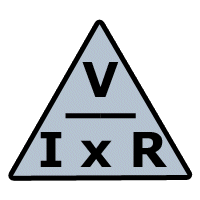ohm's lawOHM'SLAW FACTOR WHAT? SYMBOL UNIT VOLTAGE - after Alessandro Volta - electrical pressure - energy potential - voltage is the difference in potential energy between two points in a circuit VOLTAGE V VOLTAGE V volts a measure of potential energy (E) CURRENT - discovered by Ampere - rate of flow of electrons - flow of current in a closed circuit due to application of a voltage - measured in Coulombs per sec - electrons flow from negative to positive * 1 Coulomb is 6.24 x 1018  electrons I Intensity of current flow CURRENT A amps - the rate of current flow RESISTANCE - by Simon Ohm - opposition to current flow - indicates electrical energy is being converted into another energy form R resistance b In AC it is called Impedance Z = XL-inductive reactance XC - capacitive reactance ......ohms measure of the opposition to current flow

 Basic Ohm's Law  - states that V= I x R I = V/R R = V/I Example:  If a light circuit has a source voltage of 120 V and a heater load of 6 ohms then the total current flowing would be ::                                                             I = V/R   = 120V /6 ohms = 20 A (amps) V = I x R  120 V R = V/I = 6 ohms I = V/R = 20 A